Conversion of Galvanometer to Ammeter

# Materials required:

• Galvanometer
• Cell
• Rheostat
• Ammeter of desired range
• Resistance wire
• Key
• Screw gauge

# Real Lab Procedure:

• The shunt resistance required to convert the galvanometer into ammeter of range I is calculated using the formula,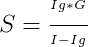G- the resistance of the galvanometer.
I- the range of desired ammeter
Ig = nk, the current required for full scale deflection in the galvanometer,
where, n- total number of divisions in the galvanometer
k- the figure of merit of the galvanometer.
Then, the length of the wire required for shunt can be calculated using the formula,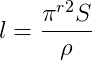Where, ρ- the resistivity of material of the wire
r- the radius of the wire, which can be measured using a screw gauge.

• Cut the resistance wire at a length of (l+2) cm.
• Make two marks near the ends of the wire so that the distance between the marks is exactly l cm.
• The wire is now connected to the terminals of the galvanometer so that the marks are just outside the terminals of the galvanometer.
• The galvanometer with the shunt connected across its terminals is the converted ammeter of the desired range.
• Connections are made as shown in the circuit diagram.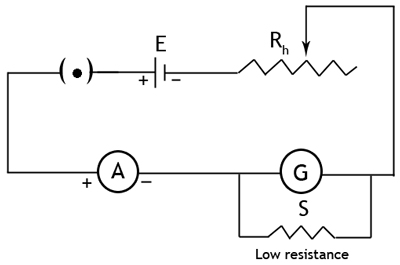• The galvanometer with shunt resistance is connected in series to a battery through an ammeter, key and rheostat.
• Insert the key.
• Adjust the rheostat and set the current reading I of the given ammeter at a particular value.
• The reading of the galvanometer Ig’ is noted. Now, the current through the converted ammeter is calculated using the relation,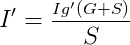• The error of the converted ammeter is calculated as I – I’.
• Repeat the experiment by changing the rheostat resistance.
• A graph can be drawn with (I – I’) along Y-axis and I’ along X-axis. This is called the correction graph.
• Thus, the converted ammeter is verified with an ammeter of the same range and a correction graph is obtained.

# Simulator procedure (as performed through the Online Labs):

• Select the ammeter range (I) from the drop down list.
• Select the galvanometer resistance (G) from the drop down list.
• Select the galvanometer range (n-total number of divisions on the galvanometer) from the drop down list.
• The cell voltage is fixed as 2V.
• Select the material of the wire from the drop down list.
• The radius of platinum wire is fixed as 0.1 mm and others is 0.2 mm.
• Enter the calculated value of shunt resistance (S), rounded for two digits, in the text box.
• Click on the ‘Submit’ button to verify your value.
• If the value is correct, enter the length (l) of the wire required for shunt, rounded for two digits, in the text box.
• Click on the ‘Submit’ button to verify your value.
• If the value is correct, click on the ‘Proceed’ button to go to the experiment.
• To see the circuit diagram, click on the 'Show/hide circuit diagram' check box seen inside the simulator window.
• Connections can be made as seen in the circuit diagram by clicking and dragging the mouse from one connecting terminal to the other connecting terminal of the devices to be connected.
• To insert the key, click on the ‘Insert key’ button.
• You can change the rheostat resistance using the slider.
• You can notice the corresponding ammeter and voltmeter readings.
• To redo the experiment, click on the ‘Reset’ button.

# Observations:

Resistance of the galvanometer, G = ............ohms
Figure of merit of the galvanometer, k = ............... amp./div.
Number of divisions in the galvanometer scale, n = ................
Current for full scale deflection, Ig = nk =............amp.

Desired range of the converted ammeter (I) ---------mA

Shunt resistance,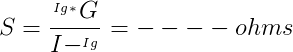Trial No. Ammeter I in amps Galvanometer readin (Ig') Current through the converted ammeterError of the converted ammeter  (I - I')

# Calculations:

Shunt resistance,Radius of the wire, r = --------cm = ------- X 10-2 m.

Resistivity of the material of the wire, ρ = --------------Ωm

Length of the wire required for shunt can be calculated using the formula,# Result:

The given galvanometer is converted into an ammeter of range 0 to ………….A by connecting a shunt resistance of …………ohms.

Cite this Simulator: